How Gage Studies Can Get it Wrong
Dr Jody Muelaner posted on May 23, 2018 |
Understanding the importance of systematic effects and how they can sometimes go undetected.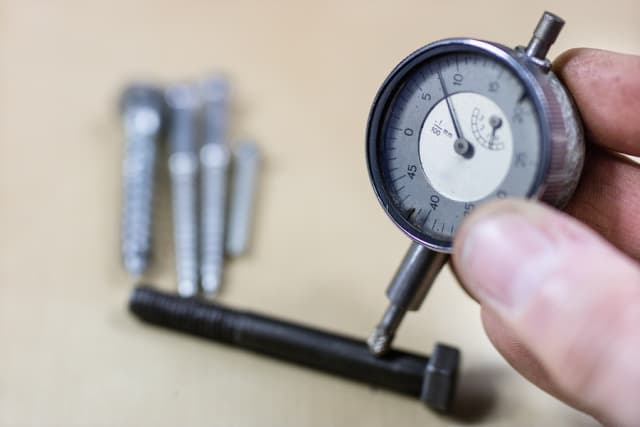In my previous articles, I explained the fundamentals of uncertainty evaluation and gage studies. I suggested that uncertainty evaluation is a more rigorous approach, but what does that mean in practical terms?

A gage study involves real measurements of a calibrated reference, so isn’t that a fairly definitive test?

Well, in this article I’m going to show you, with some real examples, how a gage study can get things wrong and how uncertainty evaluation can give a better understanding of variation and bias in a measurement process.

### Systematic Effects in Measurement

We need to start by considering systematic effects. The Guide to the Expression of Uncertainty in Measurement (GUM) talks about uncertainty arising from systematic effects while in Measurement Systems Analysis (MSA) it is the systematic errors which are discussed.

If you’re not clear on the difference between an error and an uncertainty then you should read my introduction to metrology and quality first. What is important to remember is that systematic errors are not random and may have a known cause.

When these causes are quantified the measurement result can be considered to be a function of them, the GUM refers to these quantities as influence quantities. If the influence quantity can be determined to be significantly more or less than zero then a compensation for the effect can be made. Whether or not a correction can be applied there will be still be an uncertainty about the true value of the influence quantity.

Although the GUM does not categorize uncertainties by those arising from random or systematic effects, it does assume that every effort is made to identify all significant systematic effects and then make corrections for them. This is equivalent to the concept of being in a state of statistical control or a having stable process, as used in Statistical Process Control (SPC).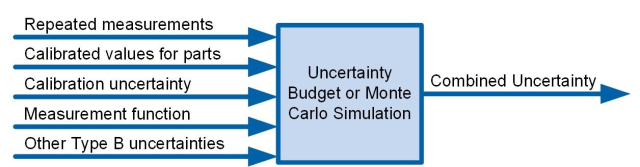An uncertainty evaluation requires repeated measurement results, calibrated values, Type B uncertainties for the calibrated references and other influences, and a measurement function which is a mathematical model of the measurement.

### Gage Studies Measure Bias

Within a Gage study, you can take a simpler approach. The mean of many measurements is compared with a reference value and the difference is the bias, also called trueness. A gage R&R ANOVA calculation is used to determine the variance components associated with repeatability and with factors such as the part and the operator. Within MSA, accuracy is defined as the combination of precision and trueness.

This approach ignores any error in the reference standard. In other words it assumes that the systematic effect of uncertainty in the reference standard is negligible. To ensure that this assumption is valid it is sometimes specified that the uncertainty (or accuracy) of the reference standard should be less than 10% of the variation in the measurement being evaluated. This is sometimes difficult to achieve and, by ignoring the uncertainty in the reference standard, a gage study will then not give a true representation of the accuracy of a measurement.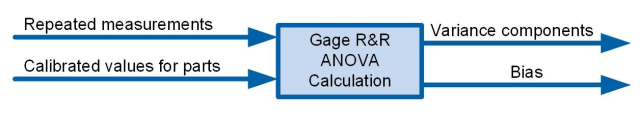A Gage R&R Study only requires the repeated measurement results and the calibrated reference values.

In the above example, where a significant uncertainty in the reference standard is not reflected in results of a gage study, an MSA practitioner should be aware that there are issues with the study. However, there are other cases where a gage study can under-estimate variation without any indication that there is an issue with the study.

The simplicity of making repeated observations and comparisons with calibrated references may give the impression that little can go wrong. However, it is this simplicity which can cause issues in certainty cases, the variation and bias observed in a set of observations is only that resulting from influences which are present during these observations.

Although a quality engineer should, in theory, consider all potential sources of variation and include these in a study, this is often not practical. In the real world, a standard Gage R&R study is usually used involving a number of parts, operators and repetitions.

The implicit assumption is that the part and the operator are the only significant reproducibility conditions. It is highly likely that there may be other significant influences, but if they are not considered during the design of the study, they may not be varied noticeably while measurements are being made.

### Significant, but hard to detect, Influences on Measurements

Let us consider the example of environmental temperature variation, which often has a significant influence on measurements. In the worst case, with no consideration at all for temperature, a gage study may be carried out when the temperature is close to nominal for all measurements. In this case there would be little variability or bias seen in the results due to temperature. During actual production conditions, however, there may be significant variations in temperature due to the time of day, roller doors being opened or due to annual seasonal variations.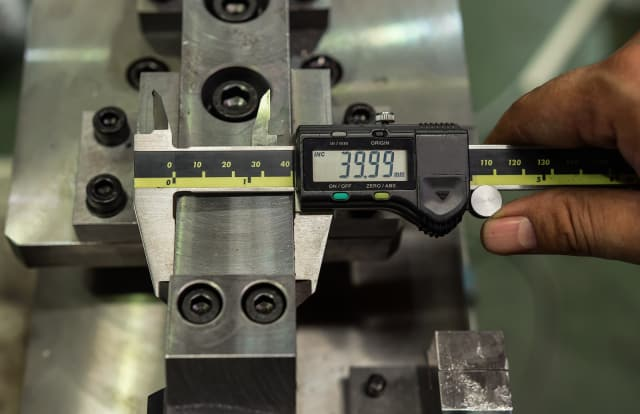You may argue that this is just bad experimental design, not an issue with the MSA approach in general. Even taking this line, it certainly does show some issues with common implementation of MSA. However, if we fully consider all of the influence quantities for a measurement we will often find that there are serious practical issues with trying to fully represent all sources of variation and bias in a gage study.

Even with simple temperature variation, the seasonal variation over a year is difficult to represent in a gage study. Also, if there are a large number of influence quantities or factors, it may not be practical to perform a well-designed experiment which is sensitive to all of them. And you can forget performing the type of full-factorial design normally used to determine the individual variance components for each factor.

### Type A and Type B Evaluations

The biggest issue with relying purely on a gage study to evaluate measurement capability is that there may be significant influences which cannot be realistically varied in the study. This is the reason for including Type B evaluations in the GUM approach, it acknowledges this limitation and provides a practical solution.

According to GUM, a Type A evaluation determines uncertainty by the statistical analysis of a series of observations, while a Type B evaluation uses any other means, but both types are then treated in the same way to determine the combined uncertainty.

In a gage study factors which would require a Type B evaluation cannot be included in a study, for example in the Total Gage R&R. Attempts are therefore made the ensure these factors have a negligible effect, such as by selecting a reference standard which has a much smaller uncertainty than the measurement being studied, but if this is not possible there is no way of including the effects in the result.

In the GUM approach we can deal with factors which may not be practical to vary, by taking the associated uncertainty from a calibration certificate, a material specification or similar.

### Systematic Effects in Gage Studies

Consider another example of how systematic effects may go unnoticed in a gauge R&R study. Let us look again at the example of temperature variation. This time, however, we will assume that the quality engineer has identified it to be a significant source of variation. The measurement process includes a correction for thermal expansion and when gage R&R studies are performed care is taken to ensure that measurements are made at a range of temperatures, representing typical variations recorded in the measurement room.

So, do you think that thermal expansion will be fully accounted for in the results of the gage R&R study?

If all of the parts used in the study come from the same batch of material, they are each likely to have a very similar coefficient of thermal expansion (CTE). The correction for thermal expansion is a function of the CTE and it therefore has an uncertainty which is a function of the uncertainty in the CTE. If we assume that for the batch used in the study the CTE is close to the nominal value specified for the material then we will not see a significant variation or bias due to uncertainty in the CTE.

However, when the batch changes, if the CTE deviates significantly from nominal then this will introduce a bias in the corrections for thermal expansion, which will vary with the temperature offset for which we are correcting.

With the new batch, if we made repeated measurements of the same part at different temperatures we would see an increased variation in the measurement result due to errors in the corrections for thermal expansion. A simple correction for thermal expansion is given by

Δ L = Δ T L α

where ΔT is the temperature offset from the nominal temperature, L is the nominal length and α is the CTE for the material.

We will assume that the actual measurements of the length and the temperature have negligible uncertainty. Considering the measurement of a 500mm length with temperature offsets of up to 5 degrees C, if the true CTE of the part, αTrue is 18 10-6/C we would see errors in the uncorrected measurement of up to 45 micrometres since

ΔL = ΔT L αTrue  = 5ºC × 0.5m × 18 10-6 / C = 45 µm

If the specified CTE for the part, αSpec is 12 10-6/C then the corrected measurements would not fully correct for the effects of thermal expansion and we would see residual errors of up to 15 micrometres since

ΔL = ΔT L αTrue - ΔT L αspec  = 5ºC × 0.5m × ( 18 10-6/ C - 12 10-6 / C ) = 15µm

It is virtually impossible to accurately quantify an effect like this using a gage study. It can, however, be easily considered as a Type B uncertainty by simply taking the uncertainty in the CTE from the material specification.

The most reliable and practical approach is a hybrid methodology involving a combination of gage studies and uncertainty evaluation as recommended in VDA5. I’ll talk more about this in a future post.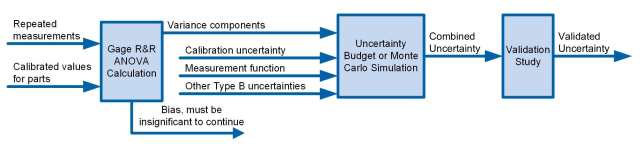A hybrid approach can be taken in which a gage R&R study is used to quantify variance components and these are then combined with Type B uncertainties in an uncertainty budget, finally a validation study can be used to validate the uncertainty budget.

### Systematic Effects in Statistical Process Control

Similar issues can also arise in statistical process control (SPC). This occurs when a systematic effect acts on both the output of a manufacturing process and measurements of that output. In this case, an error in the process output will be matched by a very similar error in the measurement, effectively hiding the effect in the data. It will only be an issue if the measurement is not capable, i.e., if the uncertainty of the measurement not small enough with respect to the process tolerance.

Therefore, if the systematic effect has been correctly accounted for in the uncertainty evaluation it should not be an issue. If, however, a gage study has been used to evaluate measurement capability then it is possible that a very large systematic effect may have been ignored, as explained above.

The potential implications of this are best understood through an example, so let’s return to thermal expansion.

This time, an aluminium part is produced on a steel machine tool and then measured using a steel gage. I will consider the effect of different gage materials. For simplicity, I will again assume that the only significant source of variation in the machining process is thermal expansion and that all other uncertainties are negligible. Therefore, the true length of the part at the time that it is produced will be the nominal (specified) length of the part plus the thermal expansion of the machine, given by

LM(T + ΔT) = L + ΔT L αM

where LM(T+ΔT) is the true length of the part at the time that it is machined at a temperature of T + ΔT, T is the reference temperature, normally 20°C, and ΔT is the temperature offset, L is the nominal length of the part and αM is the CTE for the machine.

At the reference temperature, of 20 degrees C, the part will have a true length LP(T) given by

Lp(T) = LM(T + ΔT) - ΔT L αp

where αP is the CTE for the part.

Substituting for LM(T+ΔT) gives the part length as

LP(T) = L + ΔT L (αM - αp)

If we assume that the temperature of the part does not change between machining and measuring then the measurement result is given by

LG = LM(T + ΔT) - ΔT L αG

where αG is the CTE of the gauge

Substituting for LM(T+ΔT) gives

LG = L + ΔT L (αM - αG)

Therefore, if the CTE of the machine is equal to the CTE of the gage then the gage will always give the nominal value, and any errors in the part due to thermal expansion will not be detected. If the coefficients are similar then variation will be underestimated and if the CTE of the gage is less than the CTE of the machine then the sign of the error will be reversed.

If, for example, the machine tool and gage are both made from tool steel but the part is aluminium then the thermal errors will not be visible in the measurement results. If the machine tool is made from tool steel, the part from aluminium and the gage from invar steel then the errors will be reversed—this could cause big problems if you are trying to apply a correction!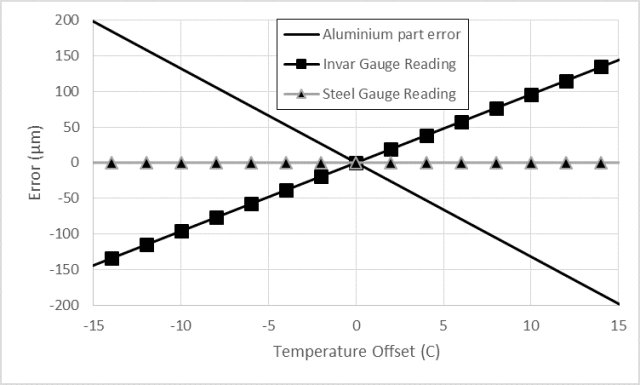Thermal expansion errors for a 1,200 mm aluminium part measured with tool steel or invar gages.
I hope these examples have given you a clearer understanding of the importance of considering systematic effects. Gage studies are a great tool but they can sometimes miss these effects.

A little time spent considering the uncertainty budget for a measurement can go a long way to detecting potential issues of this type. Ideally a hybrid approach should be taken in which gage studies are used as part of an uncertainty evaluation.

I’ll look at this in more details next time.Dr. Jody Muelaner’s 20-year engineering career began in machine design, working on everything from medical devices to saw mills. Since 2007 he has been developing novel metrology at the University of Bath, working closely with leading aerospace companies. This research is currently focused on uncertainty modelling of production systems, bringing together elements of SPC, MSA and metrology with novel numerical methods. He also has an interest in bicycle design. Visit his website for more information.
 Recommended For You